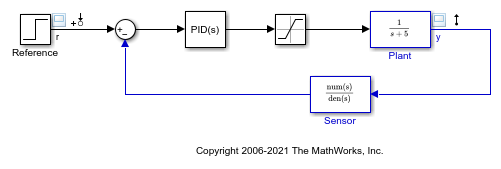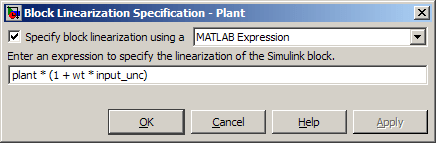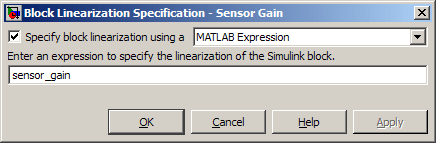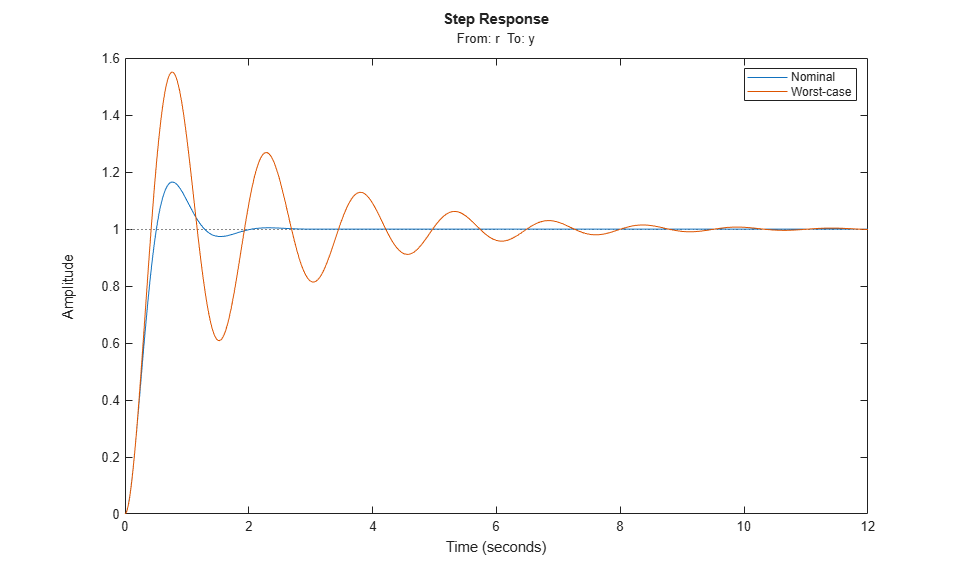Documentation

### This is machine translation

Mouseover text to see original. Click the button below to return to the English version of the page.

Note: This page has been translated by MathWorks. Click here to see
To view all translated materials including this page, select Country from the country navigator on the bottom of this page.

## Linearization of Simulink Models with Uncertainty

This example shows how to compute uncertain linearizations using Robust Control Toolbox™ and Simulink® Control Design™. There are two convenient workflows offered depending on how Simulink is used. The resulting uncertain linearizations are in the form of the uncertain state space (USS) data structure in the Robust Control Toolbox, which can be used by the analysis functions in Robust Control Toolbox.

### Introduction

The graphical user interface in Simulink is a natural environment to model and simulate control systems. Using the linearization capabilities in Simulink Control Design and the uncertainty elements in Robust Control Toolbox, you can specify uncertainty on specific blocks in a Simulink model and then extract an uncertain linearized model.

In this example, the performance of a PID controller is examined in the presence of uncertainty. There are two approaches available to compute linearizations of uncertain systems. Each of the approaches is designed to meet different needs when working in Simulink. These approaches are summarized in the following sections.

### Approach #1: Using Uncertain State Space Blocks

This first approach is most applicable when you are already using Uncertain State Space blocks as part of your control system design process in Simulink. As shown in the example Robustness Analysis in Simulink, the Uncertain State Space block in Robust Control Toolbox lets you specify uncertainty in a Simulink model.

In the following example, both the plant and sensor dynamics are uncertain. The uncertainty on the plant dynamics includes:

• Real pole `unc_pole` whose location varies between -10 and -4

• Unmodeled dynamics `input_unc` (25% relative uncertainty at low frequency rising to 100% uncertainty at 130 rad/s).

```unc_pole = ureal('unc_pole',-5,'Range',[-10 -4]); plant = ss(unc_pole,5,1,0); wt = makeweight(0.25,130,2.5); input_unc = ultidyn('input_unc',[1 1]); ```

The uncertain sensor dynamics are defined to be

```sensor_pole = ureal('sensor_pole',-20,'Range',[-30 -10]); sensor = tf(1,[1/(-sensor_pole) 1]); ```

The `rct_ulinearize_uss` model uses Uncertain State Space blocks (highlighted in blue) to model this uncertainty:

```mdl = 'rct_ulinearize_uss'; open_system('rct_ulinearize_uss') ```This Simulink model is ready to compute an uncertain linearization. The linear model has an input at the reference block `rct_ulinearize_uss/Reference` and an output of the plant `rct_ulinearize_uss/Uncertain Plant`. These linearization input and output points are specified using Simulink Control Design. The linearization points are found using the following command:

```io = getlinio(mdl); ```

The uncertain linearization is computed using the command `ulinearize`. This command returns an uncertain state space (USS) object that depends on the uncertain variables `input_unc`, `sensor_pole`, and `unc_pole`:

```sys_ulinearize = ulinearize(mdl,io) ```
```sys_ulinearize = Uncertain continuous-time state-space model with 1 outputs, 1 inputs, 5 states. The model uncertainty consists of the following blocks: input_unc: Uncertain 1x1 LTI, peak gain = 1, 1 occurrences sensor_pole: Uncertain real, nominal = -20, range = [-30,-10], 1 occurrences unc_pole: Uncertain real, nominal = -5, range = [-10,-4], 1 occurrences Type "sys_ulinearize.NominalValue" to see the nominal value, "get(sys_ulinearize)" to see all properties, and "sys_ulinearize.Uncertainty" to interact with the uncertain elements. ```

This concludes the first approach. Close the Simulink model:

```bdclose(mdl) ```

### Approach #2: Using Built-in Simulink Blocks

The second approach uses the Simulink Control Design user interface for block linearization specification to specify uncertainty for linearization. The block linearization specification feature in Simulink Control Design allows any Simulink block to be replaced by either a gain, an LTI object, or a Robust Control Toolbox uncertain variable. This approach is best suited when working with models that do not use the Uncertain State Space block. The primary advantage of this approach is that the specification of the uncertainty does not impact any other operation in Simulink such as simulation.

A modified version of the original model using only built-in Simulink blocks is shown below.

```mdl = 'rct_ulinearize_builtin'; open_system(mdl); ```By right clicking on the `rct_ulinearize_builtin/Plant` block and selecting the menu item Linear Analysis->Specify Linearization, you can specify what value this block should linearize to. If you enter the expression `plant*(1+wt*input_unc)` in the dialog box shown below, the "Plant" block will linearize to the corresponding uncertain state-space model (USS object).Similarly, you can assign the uncertain model `sensor` as linearization for the block `rct_ulinearize_builtin/Sensor Gain`.You can now linearize `rct_ulinearize_builtin` using the Simulink Control Design command `linearize`:

```io = getlinio(mdl); sys_linearize = linearize(mdl,io) ```
```sys_linearize = Uncertain continuous-time state-space model with 1 outputs, 1 inputs, 5 states. The model uncertainty consists of the following blocks: input_unc: Uncertain 1x1 LTI, peak gain = 1, 1 occurrences sensor_pole: Uncertain real, nominal = -20, range = [-30,-10], 1 occurrences unc_pole: Uncertain real, nominal = -5, range = [-10,-4], 1 occurrences Type "sys_linearize.NominalValue" to see the nominal value, "get(sys_linearize)" to see all properties, and "sys_linearize.Uncertainty" to interact with the uncertain elements. ```

The resulting model is an uncertain state-space (USS) model equivalent to the uncertain linearization computed using the first approach.

### Leveraging the Uncertain Linearization Result

Both linearization approaches produce an uncertain state-space (USS) object which can be analyzed with standard Robust Control Toolbox commands. In this example, this USS model is used to find the worst-case gain of the linearized closed-loop response.

```[maxg,worstun] = wcgain(sys_linearize); ```

The resulting worst-case values for the uncertain variables can then be used to compare against the nominal response. This comparison indicates that the PID performance is not robust to the plant and sensor uncertainty.

```sys_worst = usubs(sys_linearize,worstun); step(sys_linearize.NominalValue,sys_worst) legend('Nominal','Worst-case'); ```This concludes the example. Close the Simulink model:

```bdclose(mdl); ```
##### SupportGet trial now